# Geometry Vocabulary Terms with Pictures Flashcards

Set Details Share
created 12 years ago by jingle
24,435 views
Common geometry terms that make learning geometry easier. Includes a picture for each term.
Subjects:
math, geometry, vocabulary
Page to share:
Embed this setcancel
COPY
code changes based on your size selection
Size:
X

1

Point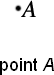An exact location in space, usually represented by a dot

2

Angle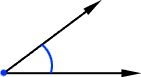A figure formed by two rays that have a common endpoint

3

Vertex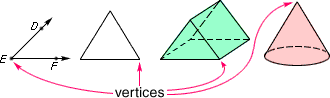The point where two or more rays meet; the point of intersection of two sides of a polygon; the point of intersection of three or more edges of a solid figure; the top point of a cone; the plural of vertex is vertices

4

Circle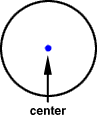A closed plane figure with all points on the figure the same distance from the center

5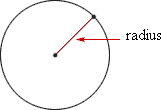A line segment with one endpoint at the center of a circle and the other endpoint on the circle

6

Obtuse Triangle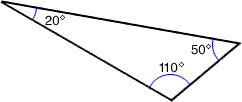A triangle that has one obtuse angle

7

Polygon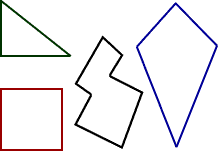A closed plane figure formed by three or more line segments

8

Square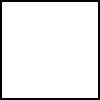A rectangle with 4 equal sides

9

Pentagon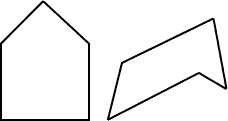A polygon with five sides

10

LineA straight path in a plane that goes on forever in opposite directions

11

Right AngleAn angle formed by perpendicular lines, line segments, or rays and with a measure of 90°

12

Parallel Line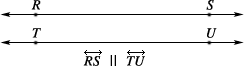Lines in a plane that never intersect

13

Circumference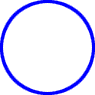The distance around a circle

14

Triangle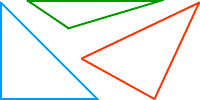A polygon with three sides

15

Equilateral Triangle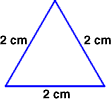A triangle with three congruent sides

16

Parallelogram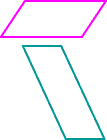A quadrilateral whose opposite sides are parallel and congruent

17

Trapezoid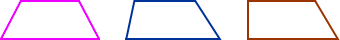A quadrilateral with one pair of parallel sides

18

Closed Figure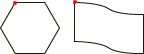A figure that begins and ends at the same point

19

Line Segment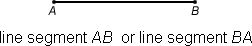A part of a line that includes two points, called endpoints, and all of the points

20

Acute AngleAn angle that has a measure less than a right angle (less than 90°)

21

Perpendicular LinesTwo lines that intersect to form four right angles

22

Chord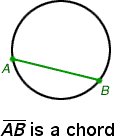A line segment with its endpoints on a circle

23

Right Triangle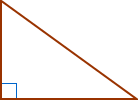A triangle with one right angle

24

Isosceles Triangle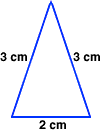A triangle with two congruent sides

25A polygon with four sides

26

Hexagon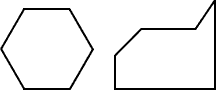A polygon with six sides and six angles

27

Ray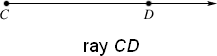A part of a line, with one endpoint, that continues without end in one direction

28

Obtuse Angle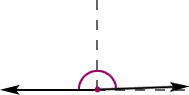An angle whose measure is greater than 90° and less than 180°

29

Intersecting LinesLines that cross at exactly one point

30

Diameter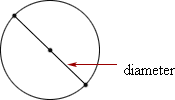A line segment that passes through the center of
a circle and has its endpoints on the circle

31

Acute Triangle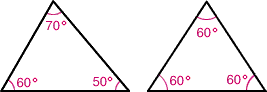A triangle in which all three angles are acute

32

Scalene Triangle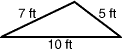A triangle with no congruent sides

33

RectangleA parallelogram with four right angles

34

Octagon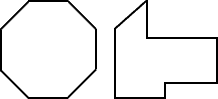A polygon with eight sides